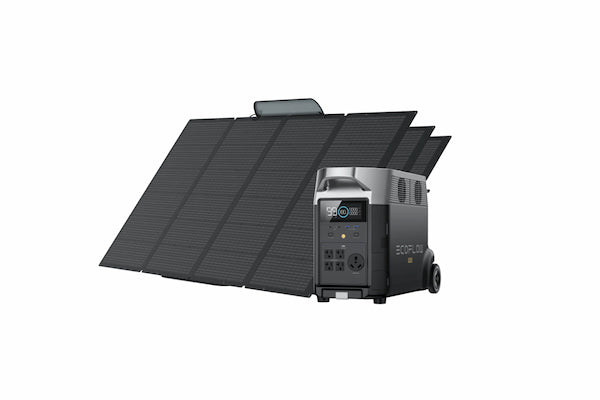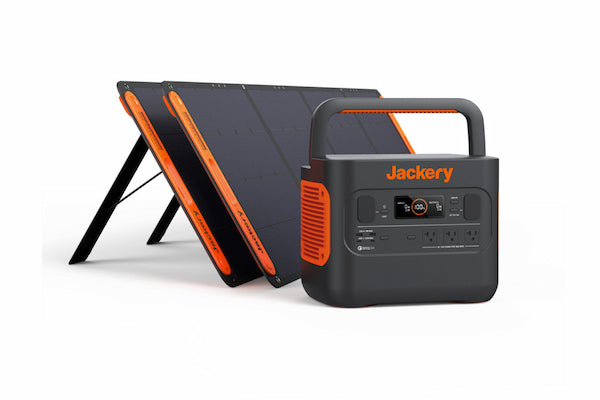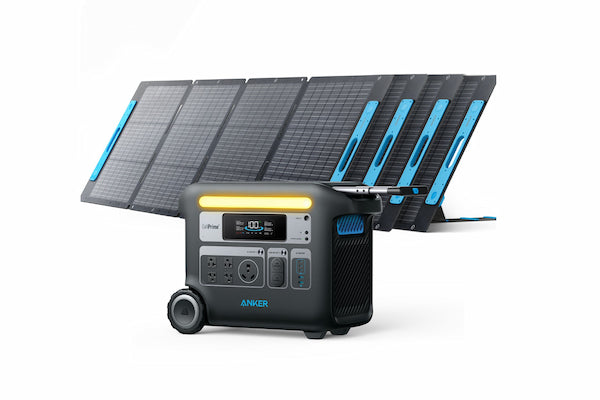# From Volts to Watts: A Guide into Solar Power's Essential Units

SolarJerry Lee

The world of solar energy can be filled with perplexing electrical terminology. However, gaining a clear understanding of these terms is vital for comprehending how solar systems function. Units of measurement such as volts, watts and amps play crucial roles in solar power, and familiarizing yourself with their meanings empowers you to make informed decisions about your solar system. In this article, we will unravel the complexities of volts and watts, delve into important electrical units like amps, watt-hours, and kilowatt-hours, and provide practical formulas for converting volts to watts and vice versa. Whether you're a solar enthusiast or a curious learner, this guide will equip you with the knowledge you need to navigate the world of solar power effectively.## Introduction to Some Main Electrical Units

First of all, let's explore the definition of some key electrical units that form the foundation of electricity and what they mean when comes to solar power.

### What Is Watt?

A watt is a unit of power in the International System of Units (SI), named after the Scottish engineer James Watt. It measures the rate at which energy is consumed or produced. Specifically, one watt is equal to one joule of energy per second. It is commonly used to quantify the electrical power consumed or generated by devices like light bulbs and appliances. The wattage rating of a device indicates its power requirements or output.

Solar panels are rated in watts (W), indicating their power output under standard test conditions, such as specific sunlight intensity. For instance, a solar panel rated at 300 watts can generate up to 300 watts of electricity under optimal conditions.

### What Is Volt?

A volt is a unit that measures electrical potential difference or the "push" of electric power, named after the Italian physicist Alessandro Volta. It represents the intensity of the force that makes electric charges move in a circuit. It is commonly used to express the voltage of electrical systems and devices, such as the outlets at homes.

In the context of solar energy, volts describe the electrical output of solar panels and the requirements of solar power systems. It helps ensure compatibility and efficient energy transfer in solar installations.

### Other Important Electrical Units:

• Amps, short for amperes, measure electric current, indicating the flow rate of electric charges in a circuit.
• Watt-hours (Wh) measure electricity consumption or battery capacity, representing the total energy consumed or produced over time. They are calculated by multiplying power consumption in watts by the duration in hours. For example, if a device uses 10 watts for 5 hours, it consumes 50 watt-hours of energy.
• Kilowatts (kW) are equivalent to 1,000 watts, and one kilowatt-hour (kWh) is consuming or producing 1,000 watt-hours of energy within an hour. kWh is commonly used on electricity bills to indicate energy consumption or battery storage.

A solid grasp of these units is essential for a comprehensive understanding of solar energy.

## Volts VS. Watts: What's the Difference?

Many people often get confused and wonder about the difference between volts and watts. Volts and watts are two different units of measurement used to describe electricity. Volts measure the potential difference between two points in an electric circuit, while watts measure the rate at which energy is transferred through a circuit.

A simple analogy to help understand the difference between volts and watts is to think of water flowing through a pipe. Volts would be like the pressure of the water, while watts would be like the flow rate of the water. Volts measure the force or electrical pressure of a system, while watts measure the amount of energy being consumed by that system.

Let's say you have an electric fan that consumes 50 watts of electrical power. The fan always consumes 50 watts of power, but the required voltage can vary depending on the electrical system it's connected to.

## How to Convert Volts to Watts?

Understanding the conversion from volts to watts can greatly simplify and enhance your solar or electrical calculations. To calculate volts to watts, follow these steps:

• Determine the Voltage (Volts): Identify the voltage value in your circuit. This could be the voltage of a power source, such as a battery or outlet, or the voltage across a specific component in the circuit.
• Determine the Current (Amps): Determine the current flowing through the circuit element that corresponds to the voltage you're working with. This could involve measuring the current with a multimeter or using a known current value if you're dealing with a specific device.
• Apply the Formula: Plug the voltage value (in volts) and the current value (in amps) into the formula: Watts (W) = Volts (V) × Current (I)
• Calculate the Result: To convert volt ampere to watt, multiply the voltage by the current to get the power in watts. The resulting value will be the power consumed or produced by the circuit element.

Keep in mind that the formula assumes ideal conditions and doesn't account for factors like efficiency, power factor, or losses in the circuit.

## How to Convert Watts to Volts?

To convert watts to volts, you can simply rearrange the volts to watts formula. The watts to volts formula is:

The formula for converting watts to volts is:

Volts (V) = Watts (W) / Current (I)

You can also use a watts to volts calculator to convert watts to volts.

## Best Solar Generator

Solar generators convert sunlight into electrical energy using portable solar panels and a power station. The voltage of a solar generator determines its electrical pressure, while the wattage indicates its power capacity. These factors are crucial as they determine the compatibility and number of devices that can be powered simultaneously by the solar generator.

With a grasp of volts and watts, you can now appreciate why the following models are considered top-notch in the market.

### 1. EcoFlow Solar Generator DELTA ProThe EcoFlow Solar Generator DELTA Pro is an advanced power solution designed to meet your high-energy demands. Some of its features are as follows:

• AC Output: It provides a total of five AC outlets with a combined maximum power of 3600W (7200W surge), making it ideal for simultaneously running multiple high-power devices and appliances, including air conditioners, refrigerators and electric heaters.
• USB-C Output: With two USB-C outputs capable of delivering up to 100W (5V, 9V, 12V, 15V, 20V up to 5A), it enables fast charging of USB-C devices, including laptops and smartphones.
• Solar Charging Input: It supports a solar charging input with a maximum power of 1600W, ensuring efficient charging directly from solar panels.

### 2. Jackery Solar Generator 2000 ProThe Jackery Solar Generator 2000 Pro is an embodiment of versatile power generation tailored to modern needs. Let's delve into the reasons behind its remarkable performance:

• AC Output: Featuring three AC outputs with a voltage of 120V, a frequency of 60Hz, and a maximum power of 2,200 watts (4,400 watts peak), it provides ample power to simultaneously run multiple household appliances and devices, like televisions and a range of lights.
• USB-C Output: With two USB-C outputs delivering up to 100W (5V, 9V, 12V, 15V, 20V up to 5A) of power, it caters to the charging needs of high-wattage devices like laptops.
• Car Port: Equipped with a 12V⎓10A car port, it allows for convenient charging of car accessories and other 12-volt devices.

### 3. Anker SOLIX F2000 Solar GeneratorThe Anker SOLIX F2000 Solar Generator represents a groundbreaking advancement that prioritizes customized power remedies. Let's explore the exceptional features that set it apart:

• AC Output: Its inverter mode offers an AC output with a voltage of 120V and a maximum power of 2400 watts. This ensures a dependable power supply for a wide range of devices and small appliances, including popular kitchen appliances like blenders, coffee makers, and toasters.
• USB-C Output: With power delivery at multiple voltages (5V, 9V, 15V, 20V), and a maximum power output of 100W per port, it ensures efficient charging of high-power USB-C devices, such as laptops.
• Charging Inputs: Supporting an AC input with a voltage of 120V and a maximum power of 1440W, as well as an XT60 input allowing a wide range of charging options (11V to 60V), it offers versatility and adaptability for different power sources.

## Final Thoughts

In this comprehensive guide, you must have gained a deep understanding of volts and watts in the context of solar energy. Understanding these fundamental units of measurement empowers you to make informed decisions about your solar system, optimize energy production, and ensure compatibility with electrical devices. Whether you're considering solar installations for your home or exploring portable solar generators for on-the-go power, the grasp of volts and watts will be invaluable.

## Recommended Posts

1.2.3.4.5.6.7.8.9.10.11.12.13.14.15.16.17.18.